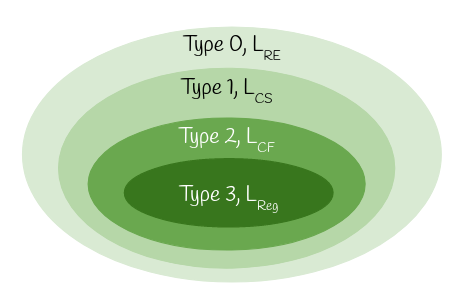# Chomsky Hierarchy

American linguist Noam Chomsky defined in 1956 a classification of languages in terms of four levels of complexity. This four-level hierarchy, called the Chomsky hierarchy, corresponds to four classes of machines .

The following table summarizes each of Chomsky's four types of grammars, the class of language it generates, and the type of machine that recognizes it.

Grammar Language Machine
Type 0
Unrestricted
grammar
$$L_{\texttt{RE}}$$
Recursively enumerable language
Turing machine (TM)
Type 1
Context-sensitive
grammar
$$L_{\texttt{CS}}$$
Context-sensitive language
Linear-bounded automaton (LBA)
Type 2
Context-free
grammar
$$L_{\texttt{CF}}$$
Context-free language
Pushdown automaton (PDA)
Type 3
Regular
grammar
$$L_{\texttt{Reg}}$$
Regular language
Finite automaton (DFA/NFA)

Each language of type $$i$$ is a proper subset of the family of type $$i − 1$$. The following diagram exhibits the relationship clearly:## Reference

•  Linz, Peter. An Introduction to Formal Languages and Automata, 6th Edition. Jones & Bartlett Learning, 2016. ISBN: 9781284077247